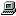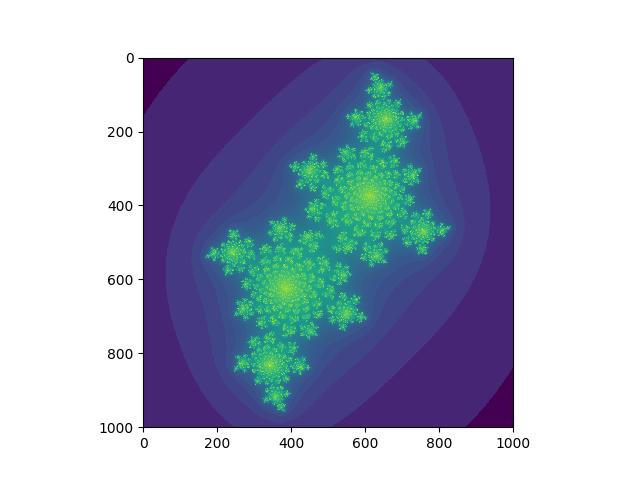# NaNg's blog

25 object(s)# 加速Python

C++的作者B.Stroustrup 说过世界上只有两种语言，一种经常有人对它抱怨，还有一种根本没人用(比如___，免得我被打请大家自行填空_(┐「ε:)_ )。

Python最经常被人抱怨的就是慢（大概比c要慢40倍，的确是挺感人的…具体数据可以参考这里）和它的gil（没有真正的线程这一点是经常遭到诟病的地方）。的确，现在cpu已经很快很快了，对于Python这样一门表达能力超强的语言，我们在一定程度上可以对它的速度有所忍受，并且对于网络应用来说python通过异步来实现并发也是可以非常快的。但Python 有时也会被用来做一些计算密集型的工作，这个时候就需要一些办法来加速了，现在看来有一大票办法来加速Python，并且其中有一些能够一定程度上解决gil的问题，最近我学习了其中几种，这里就结合一个例子来简单谈一下感受。

## 一个计算密集型过程例子：julia set

julia set 与 mandelbrot set 一样，是一种分形结构，它能由极其简单的规则所描述，但产生出的图形却十分的迷人与复杂。julia set的计算规则真的非常简单，以便理解后面的代码我先说明一下，已经理解的朋友请跳过这一部分。 julia set可以由这个复数迭代公式来描述，其中z和c都是复数，现在我们把复数空间对应到一个二维平面上，比如实数部分对应于x轴，虚数部分对应y轴，然后如果我们固定一个c的值并且对这个二维中的每一个点使用上面的公式进行迭代，会发现有的点经多次迭代后会收敛到某一范围内，另一些则会无限增大，如果我们把所有收敛的点在这个二维平面上画出来，我们将会的到非常奇妙的分形图案，并且改变c的值，图案也会发生变化，相关的图片可以看看julia set的维基页面。### pure Python

``````# jl.py
# calc_julia pure python edition

import numpy as np

def escape(z, c, z_max, n_max):
i = 0
while (z.real**2 + z.imag**2 < z_max**2) and i < n_max:
z = z*z + c
i += 1
return i

def calc_julia(resolution, c, bound=1.5, z_max=4, n_max=1000):
step = 2.0 * bound / resolution
counts = np.zeros((resolution + 1, resolution + 1), dtype=np.int32)
for i in range(resolution + 1):
real = -bound + i * step
for j in range(resolution + 1):
imag = -bound + j * step
z = real + imag * 1j
counts[i, j] = escape(z, c, z_max, n_max)
return np.asarray(counts)
``````

``````In : import matplotlib.pyplot as plt
In : import numpy as np
In : from jl import calc_julia
In : jls = calc_julia(1000, -0.4+0.6j)
In : plt.imshow(np.log(jls));plt.show()
``````### numba

numba大概是使用起来最简单的计算加速办法了吧，只需要在函数上面加一个小小的装饰器，numba就会把这个函数编译成LLVM的IR码从而达到jit的加速效果。我们来试一下。

``````# jl.py
# calc_julia numba edition

import numpy as np
from numba import jit

@jit
def escape(z, c, z_max, n_max):
i = 0
while (z.real**2 + z.imag**2 < z_max**2) and i < n_max:
z = z*z + c
i += 1
return i

@jit
def calc_julia(resolution, c, bound=1.5, z_max=4, n_max=1000):
step = 2.0 * bound / resolution
counts = np.zeros((resolution + 1, resolution + 1), dtype=np.int32)
for i in range(resolution + 1):
real = -bound + i * step
for j in range(resolution + 1):
imag = -bound + j * step
z = real + imag * 1j
counts[i, j] = escape(z, c, z_max, n_max)
return np.asarray(counts)
``````

### PyPy

pypy是一个jit的Python解释器实现，不需要修改代码就能执行，也相对比较方便，但是毕竟它已经脱离了CPython的生态，配置起来比起numba那样直接一条pip指令就能安装还是稍微麻烦一点，并且还有好多Cython中的库在这里也是没有的。把上面纯Python的代码用PyPy运行一遍,耗时0.44s，效果很可观，甚至比numba还要快一点。

### Cython

Cython即带有C类型的Python，通过引入C类型把Cython代码编译成高效的C代码，相当于使用类型信息对Python代码的“蒸馏”，并且“蒸馏”的粒度是可控的，纯Python代码在Cython中完全是合法的，只是引入越多的类型信息编译出来的代码就越高效，并且还能用来方便地包装C代码和使用C库，可以说Cython是这些方案之中最强大最成熟的一种，很多大型项目诸如Scipy、Pandas、sklearn中都大量使用了Cython。它用来写库是一种非常好的选择，对于Python用户来说Cython是非常值得学习的，如果感兴趣的话，有一本很好的讲Cython的书Cython A Guide for Python Programmers可以找来阅读一下，网上很容易找到电子版。以下的计算julia set的Cython代码就来源于这本书。

``````# jl.pyx
# calc_julia cython edition

from cython import boundscheck, wraparound
import numpy as np

cdef int escape(double complex z,
double complex c,
double z_max,
int n_max) nogil:
cdef:
int i = 0
double z_max2 = z_max * z_max
while norm2(z) < z_max2 and i < n_max:
z = z * z + c
i += 1
return i

cdef inline double norm2(double complex z) nogil:
return z.real * z.real + z.imag * z.imag

@boundscheck(False)
@wraparound(False)
def calc_julia(int resolution, double complex c,
double bound=1.5, double z_max=4.0, int n_max=1000):
cdef:
double step = 2.0 * bound / resolution
int i, j
double complex z
double real, imag
int[:, ::1] counts
counts = np.zeros((resolution + 1, resolution + 1), dtype=np.int32)
for i in range(resolution + 1):
real = -bound + i * step
for j in range(resolution + 1):
imag = -bound + j * step
z = real + imag * 1j
counts[i, j] = escape(z, c, z_max, n_max)
return np.asarray(counts)
``````

``````# setup.py
from distutils.core import setup
from Cython.Build import cythonize

setup(name="julia",
ext_modules=cythonize("jl.pyx"))
``````